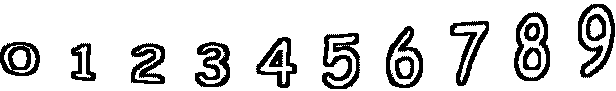5 - 5 =        6 - 2 =2 - 2 =        4 - 4 =        5 - 3 =        7 - 4 = 10 - 4 =        0 - 0 =        7 - 7 =        0 - 0 =